# Earned Value Analysis

20. Jun 2016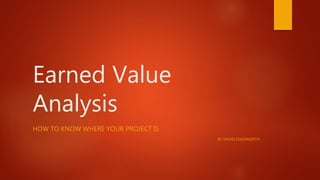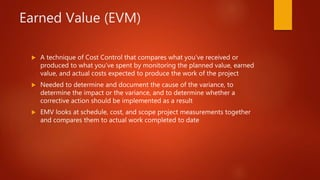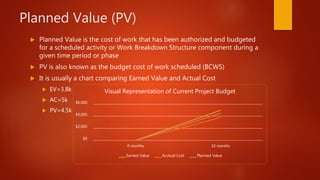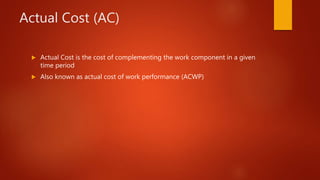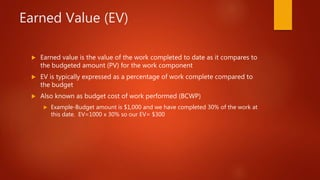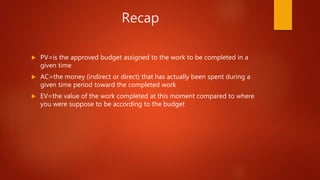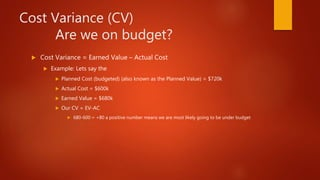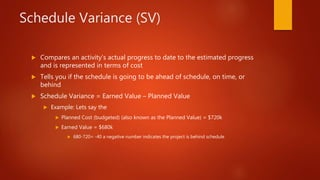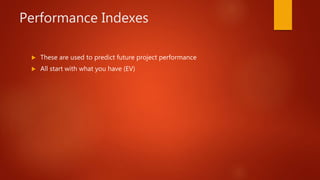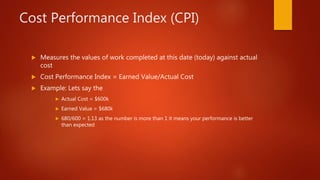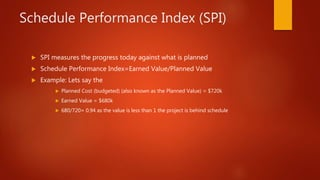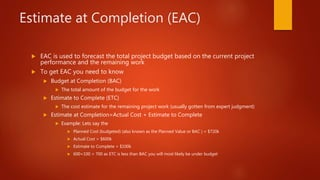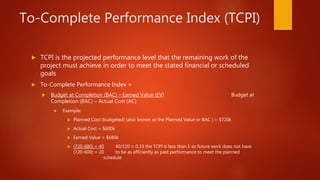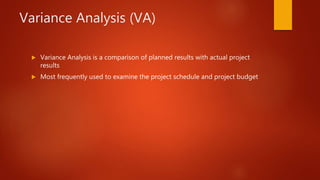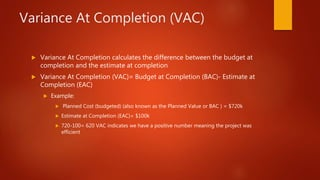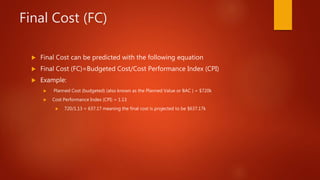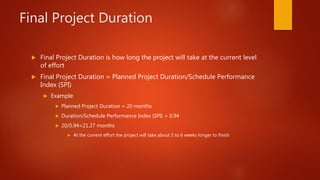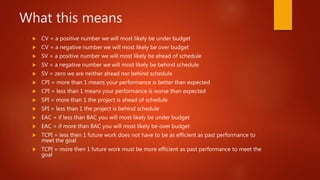1 von 18

### Earned Value Analysis

• 1. Earned Value Analysis HOW TO KNOW WHERE YOUR PROJECT IS BY DAVID EDGEWORTH
• 2. Earned Value (EVM)  A technique of Cost Control that compares what you’ve received or produced to what you’ve spent by monitoring the planned value, earned value, and actual costs expected to produce the work of the project  Needed to determine and document the cause of the variance, to determine the impact or the variance, and to determine whether a corrective action should be implemented as a result  EMV looks at schedule, cost, and scope project measurements together and compares them to actual work completed to date
• 3. Planned Value (PV)  Planned Value is the cost of work that has been authorized and budgeted for a scheduled activity or Work Breakdown Structure component during a given time period or phase  PV is also known as the budget cost of work scheduled (BCWS)  It is usually a chart comparing Earned Value and Actual Cost  EV=3.8k  AC=5k  PV=4.5k \$0. \$2,000. \$4,000. \$6,000. 0 months 10 months Visual Representation of Current Project Budget Earned Value Acutual Cost Planned Value
• 4. Actual Cost (AC)  Actual Cost is the cost of complementing the work component in a given time period  Also known as actual cost of work performance (ACWP)
• 5. Earned Value (EV)  Earned value is the value of the work completed to date as it compares to the budgeted amount (PV) for the work component  EV is typically expressed as a percentage of work complete compared to the budget  Also known as budget cost of work performed (BCWP)  Example-Budget amount is \$1,000 and we have completed 30% of the work at this date. EV=1000 x 30% so our EV= \$300
• 6. Recap  PV=is the approved budget assigned to the work to be completed in a given time  AC=the money (indirect or direct) that has actually been spent during a given time period toward the completed work  EV=the value of the work completed at this moment compared to where you were suppose to be according to the budget
• 7. Cost Variance (CV) Are we on budget?  Cost Variance = Earned Value – Actual Cost  Example: Lets say the  Planned Cost (budgeted) (also known as the Planned Value) = \$720k  Actual Cost = \$600k  Earned Value = \$680k  Our CV = EV-AC  680-600 = +80 a positive number means we are most likely going to be under budget
• 8. Schedule Variance (SV)  Compares an activity’s actual progress to date to the estimated progress and is represented in terms of cost  Tells you if the schedule is going to be ahead of schedule, on time, or behind  Schedule Variance = Earned Value – Planned Value  Example: Lets say the  Planned Cost (budgeted) (also known as the Planned Value) = \$720k  Earned Value = \$680k  680-720= -40 a negative number indicates the project is behind schedule
• 9. Performance Indexes  These are used to predict future project performance  All start with what you have (EV)
• 10. Cost Performance Index (CPI)  Measures the values of work completed at this date (today) against actual cost  Cost Performance Index = Earned Value/Actual Cost  Example: Lets say the  Actual Cost = \$600k  Earned Value = \$680k  680/600 = 1.13 as the number is more than 1 it means your performance is better than expected
• 11. Schedule Performance Index (SPI)  SPI measures the progress today against what is planned  Schedule Performance Index=Earned Value/Planned Value  Example: Lets say the  Planned Cost (budgeted) (also known as the Planned Value) = \$720k  Earned Value = \$680k  680/720= 0.94 as the value is less than 1 the project is behind schedule
• 12. Estimate at Completion (EAC)  EAC is used to forecast the total project budget based on the current project performance and the remaining work  To get EAC you need to know  Budget at Completion (BAC)  The total amount of the budget for the work  Estimate to Complete (ETC)  The cost estimate for the remaining project work (usually gotten from expert judgment)  Estimate at Completion=Actual Cost + Estimate to Complete  Example: Lets say the  Planned Cost (budgeted) (also known as the Planned Value or BAC ) = \$720k  Actual Cost = \$600k  Estimate to Complete = \$100k  600+100 = 700 as ETC is less than BAC you will most likely be under budget
• 13. To-Complete Performance Index (TCPI)  TCPI is the projected performance level that the remaining work of the project must achieve in order to meet the stated financial or scheduled goals  To-Complete Performance Index =  Budget at Completion (BAC) – Earned Value (EV) Budget at Completion (BAC) – Actual Cost (AC)  Example:  Planned Cost (budgeted) (also known as the Planned Value or BAC ) = \$720k  Actual Cost = \$600k  Earned Value = \$680k  (720-680) = 40 40/120 = 0.33 the TCPI is less than 1 so future work does not have (720-600) = 20 to be as efficiently as past performance to meet the planned schedule
• 14. Variance Analysis (VA)  Variance Analysis is a comparison of planned results with actual project results  Most frequently used to examine the project schedule and project budget
• 15. Variance At Completion (VAC)  Variance At Completion calculates the difference between the budget at completion and the estimate at completion  Variance At Completion (VAC)= Budget at Completion (BAC)- Estimate at Completion (EAC)  Example:  Planned Cost (budgeted) (also known as the Planned Value or BAC ) = \$720k  Estimate at Completion (EAC)= \$100k  720-100= 620 VAC indicates we have a positive number meaning the project was efficient
• 16. Final Cost (FC)  Final Cost can be predicted with the following equation  Final Cost (FC)=Budgeted Cost/Cost Performance Index (CPI)  Example:  Planned Cost (budgeted) (also known as the Planned Value or BAC ) = \$720k  Cost Performance Index (CPI) = 1.13  720/1.13 = 637.17 meaning the final cost is projected to be \$637.17k
• 17. Final Project Duration  Final Project Duration is how long the project will take at the current level of effort  Final Project Duration = Planned Project Duration/Schedule Performance Index (SPI)  Example  Planned Project Duration = 20 months  Duration/Schedule Performance Index (SPI) = 0.94  20/0.94=21.27 months  At the current effort the project will take about 5 to 6 weeks longer to finish
• 18. What this means  CV = a positive number we will most likely be under budget  CV = a negative number we will most likely be over budget  SV = a positive number we will most likely be ahead of schedule  SV = a negative number we will most likely be behind schedule  SV = zero we are neither ahead nor behind schedule  CPI = more than 1 means your performance is better than expected  CPI = less than 1 means your performance is worse than expected  SPI = more than 1 the project is ahead of schedule  SPI = less than 1 the project is behind schedule  EAC = if less than BAC you will most likely be under budget  EAC = if more than BAC you will most likely be over budget  TCPI = less then 1 future work does not have to be as efficient as past performance to meet the goal  TCPI = more then 1 future work must be more efficient as past performance to meet the goal